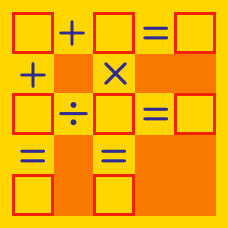Logic

Number Guessing

I am thinking of a two digit number that is divisible by 3, 4, and 5.

What number am I thinking of?

I am thinking of a three digit number.

My number is even.

The second digit is 1 larger than the first digit.

The third digit is 5 larger than the second digit.

What is the value of the second digit?

I am thinking of a 4-digit number.

The product of the digits in my number is 18.

The sum of the first three digits in my number is smaller than the last digit in my number.

How many possible numbers could I be thinking of?

I am thinking of a three-digit number.

The sum of the digits in my number is 9.

If I told you the product of the digits in my number, you would be able to uniquely identify my number.

What is the product of the digits in my number?

I am thinking of a two digit number.

My number is a perfect square.

The sum of the digits in my number is 9.

Is this enough information to uniquely identify my number?

×